0+3+0+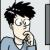0+0+0+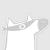0+1+0+1+4+0+0+1+0+0+1+0+0+0+0+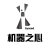0+0+0+0+1+0+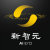0+1+0+0+3+0+0+1+0+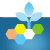0+0+0+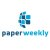【针对短文本分类的异质图注意力网络】Heterogeneous Graph Attention Networks for Semi-supervised Short Text Classification
#论文解读# #EMNLP 2019# 本文是北邮和南洋理工联合发表在EMNLP 2019的工作，论文创新性地将短文本分类问题建模为异质图（多种类型的节点和边）并提出一种端到端的异质图...全文0+0+0+0+1+0+
Top